##### Question

In: Statistics and Probability

# A study of the amount of time it takes a mechanic to rebuild the transmission for...

A study of the amount of time it takes a mechanic to rebuild the transmission for a 2010 Chevrolet Colorado shows that the mean is 8.4 hours and the standard deviation is 1.8 hours. If 40 mechanics are randomly​ selected, find the probability that their mean rebuild time is less than 8.9 hours. Round to four decimal places. A.0.9756 B.0.9605 C.0.4276 D.0.9589

## Solutions

##### Expert Solution

SOLUTION:

From given data,

A study of the amount of time it takes a mechanic to rebuild the transmission for a 2010 Chevrolet Colorado shows that the mean is 8.4 hours and the standard deviation is 1.8 hours. If 40 mechanics are randomly​ selected, find the probability that their mean rebuild time is less than 8.9 hours.

Where,

Population mean =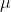= 8.4 hour

Population standard deviation =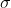= 1.8 hour

Sample mean =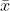= 8.9

Sample size = n = 40

Z = (-) / (/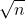)

= (8.9 - 8.4) / (1.8  /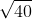)

=  0.5 / 0.2846049

= 1.76

P(X < 8.9 ) = P(Z < 1.76 )

=  0.9605

Answer : Option (B) is correct

## Related Solutions

##### A study of the amount of time it takes a mechanic to rebuild the transmission for...
A study of the amount of time it takes a mechanic to rebuild the transmission for a 1992 Chevrolet Cavalier shows that the mean is 8.4 hours and the standard deviation is 1.8 hours. Suppose 40 mechanics are randomly selected, what is the probability the average time to rebuild the transmission of a 1992 Chevrolet Cavalier for these 40 mechanics is more than 8.1 hours?
##### A study of the amount of time it takes a mechanic to rebuild the transmission for...
A study of the amount of time it takes a mechanic to rebuild the transmission for a 2005 Chevrolet Cavalier shows that the mean is 8.4 hours and the standard deviation is 1.8 hours. If 40 mechanics are randomly selected, find the probability that their mean rebuild time exceeds 8.7 hours. Group of answer choices
##### 1. A study of the amount of time it takes a recording artist to record a...
1. A study of the amount of time it takes a recording artist to record a CD shows that the mean is 11.2 hours with a standard deviation of 6.6 hours. If 40 recording artists are randomly selected, find the probability that their mean recording time is between 9.75 and 12.55 hours. Probability Statement:: ________________________ z-Score: ____________ Answer
##### The amount of time it takes for a student to complete a statistics exam is given...
The amount of time it takes for a student to complete a statistics exam is given by a random variable that is uniformly distributed between 28 and 61 minutes. A student is selected at random. Find the probability of the following events. In each case, give exact answers or round to at least three decimal places. The student’s time was between 34 and 39 minutes The student’s time was less than 34 minutes The student’s time was between 39 and...
##### Suppose you are interested in measuring the amount of time, on average, it takes you to...
Suppose you are interested in measuring the amount of time, on average, it takes you to make your commute to school. You've estimated that the average time is 35.68 minutes with a standard deviation of 6.17 minutes. Assuming that your estimated parameters are correct and the commute time is normally distributed, what is the probability that the average commute time of 11 random days is greater than 36.86 minutes? Question 8 options: 1) 0.2629 2) 0.7371 3) 0.5758 4) 0.8246...
##### An insurance company has the business objective of reducing the amount of time it takes to...
An insurance company has the business objective of reducing the amount of time it takes to approve applications for life insurance. During the current month, you collect a random sample of 30 approved policies. The total processing time for these policies can be found in the Excel data file for this assignment under the tab Insurance. a. In the past, the mean processing time was 45 days. At the 0.05 level of significance, is there evidence that the mean processing...
##### A management consultant is studying the factors affecting the amount of time that it takes system...
A management consultant is studying the factors affecting the amount of time that it takes system administrators to complete tasks. In particular, she is interested in predicting the amount of time (in minutes) that it will take administrators to complete tasks, based on a set of variables collected for the engagement. The independent variables include – the age of the administrator (“Age”), the number of months of administrative job experience (“Experience”), whether or not the administrator has taken a job...
##### 3. a) The amount of time it takes you to fall asleep is called “sleep latency”....
3. a) The amount of time it takes you to fall asleep is called “sleep latency”. Sleep latency in adults is Normal with a standard deviation of 3.5 minutes. Forty-nine healthy adults volunteered for a sleep study, where their sleep was monitored for one night. The volunteers had an average sleep latency of 17.1 minutes. i. Compute the 80% confidence interval for the parameter of interest in this study. ii. Interpret the previously computed interval in the context of the...
##### The average amount of time it takes for couples to further communicate with each other after...
The average amount of time it takes for couples to further communicate with each other after their first date has ended is 2.01 days. Is this average different for blind dates? A researcher interviewed 68 couples who had recently been on blind dates and found that they averaged 2 days to communicate with each other after the date was over. Their standard deviation was 0.291 days. What can be concluded at the the αα = 0.10 level of significance? For...
##### A medical researcher wants to investigate the amount of time it takes for patients' headache pain...
A medical researcher wants to investigate the amount of time it takes for patients' headache pain to be relieved after taking a new prescription painkiller. She plans to use statistical methods to estimate the mean of the population of relief times. She believes that the population is normally distributed with a standard deviation of 15 minutes. How large a sample should she take to estimate the mean time to within 4 minutes with 95% confidence?